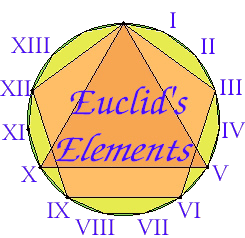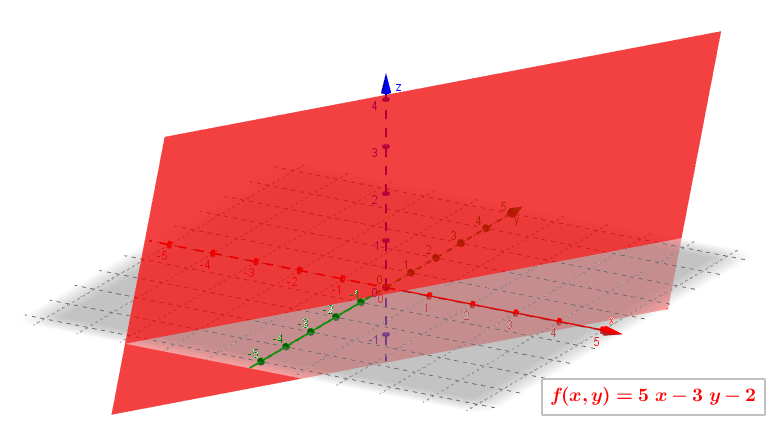## Dec. 9, 2013

Martin Flashman
¤
Humboldt State University
Arcata, CA 95521
flashman@humboldt.edu
flashman.neocities.org

#### Euclid¤Book I

A point is that which has no part.
The ends of a line are points.
A straight line is a line which lies evenly with the points on itself.
A surface is that which has length and breadth only.
The edges of a surface are lines.
A plane surface is a surface which lies evenly with the straight lines on itself.

####Descartes  ¤

The idea of this system was developed in 1637 in writings by Descartes and independently by Pierre de Fermat.
Both authors used a single axis in their treatments and have a variable length measured in reference to this axis. The concept of using a pair of axes was introduced  in 1649 by Frans van Schooten and his students.

Choosing a Cartesian coordinate system for a straight line—means choosing a point O of the line (the origin), a unit of length, and an orientation for the line.

A line with a chosen Cartesian system is called a number line.

Points in a  Euclidean plane are located by an ordered pair of cartesian coodinates, $(x,y)$.
A line in a cartesian/Euclidean plane is identified with a set of points where a point  is on the line if and only of its cartesian coordinates $(x,y)$ satisfy an "linear" equation: $Ax + By = C$.
Points in a  Euclidean space are located by an ordered triple of cartesian coodinates, $(x,y,z)$.
A plane in a cartesian/Euclidean space is identified with a set of points where a point  is on the plane if and only of its cartesian coordinates $(x,y,z)$ satisfy an "linear" equation: $Ax + By +Cz = D$.

#### ¤

The concept of "function" was coined by Gottfried Leibniz, in a 1673 letter, to describe a quantity related to a curve.
Peter Gustav Lejeune Dirichlet (in about 1837)and Nikolai Lobachevsky are traditionally credited with independently giving the modern "formal" definition of a function as a relation in which every first element has a unique second element.

A non-vertical cartesian line corresponds to a "linear function" where $y = f(x) = mx+b = Ax + B$.

A non-vertical cartesian plane corresponds to a "linear function" where $z = f(x,y) = Ax + By + C$.

The tradition is to visualize a linear function using the cartesian plane or space and the graph of the function is a line in the plane or a plane in space.

Instead of using  a graph to visualize (linear) functions, an alternative is available:

Mapping Diagrams¤

#### Example: LF.DA.0  with GeoGebra .

For more on linearity [composition and inverses] go to http://flashman.neocities.org/MD/section-2.1LF.html.

• ##### The Focus Point for a Linear Function ¤

Example: LF.DFP  with GeoGebra

“Linear” Functions with  two variables
$z=f(x,y) = Ax + By + C$

Traditional Approaches to visualizing functions of two variables:
¤
• Planar surface in cartesian space, $\{(x,y,z): z = f(x,y) = Ax +By +C\}$:
A Plane• Tables: “Addition and Multiplication Tables” ¤
• Scalar Fields: At $(x,y)$ place number $f(x,y)$.
• Level “curves”: $LC = \{ (x,y): f(x,y) = Ax+By +C= C_0\}$
A family of  parallel lines.

This is a Java Applet created using GeoGebra from www.geogebra.org - it looks like you don't have Java installed, please go to www.java.com

• Mapping Diagrams. The “linear point”.
A 3D Mapping Diagram for a Linear Function of 2 Variables ¤

# Questions?

Mapping Diagrams from A(lgebra) B(asics) to C(alculus) and D(ifferential) E(quation)s.
A Reference and Resource Book on Function Visualizations Using Mapping Diagrams

http://flashman.neocities.org/MD/section-2.1LF.html